# PHP Data Types

As PHP is a loosely types language, that is, we didn't have to tell PHP which data types the variable is. Because PHP automatically converts a variable to the correct data type, it depends on the value of the variable. Basically PHP has eight data types, can be used to construct variables in PHP scripts:

• NULL
• Integers
• Booleans
• Doubles
• Arrays
• Strings
• Resources
• Objects

## PHP Integer Data Type

PHP integer data types are simply whole numbers, without any decimal point such as 23, 3, 543, etc. Here is an example of assigning integer values to the PHP Variables:

```\$int_var = 145;
\$sum_ints = -1235 + 1235;```

Let's take a PHP integer data type program example to understand about integer data type in PHP:

```<html>
<title>PHP Integer Data Type - CodesCracker</title>
<body>
<?php
# PHP Integer Data Type Example - CodesCracker
\$integer_data_type = 10/2;
echo \$integer_data_type;
echo "<br/>";
\$integer_data_type = 10/3;
echo \$integer_data_type;
echo "<br/>";
// type casting used in below line
echo (int)\$integer_data_type;
echo "<br/>";
\$integer_data_type = 10.2/.2;
echo \$integer_data_type;
echo "<br/>";
\$integer_data_type = 10.7/.2;
echo \$integer_data_type;
echo "<br/>";
// type casting used in below line
echo (int)\$integer_data_type;
echo "<br/>";
?>
</body>
</html>```

The above PHP integer data type example code will produce the output given here: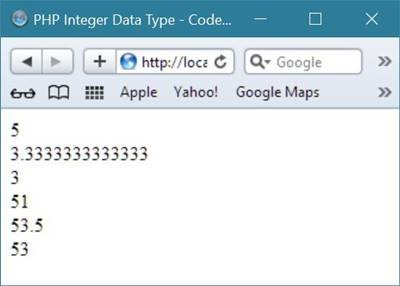As you can see here in the above PHP program, type casting is used, you will learn about type casting later in this tutorial of data type in PHP.

## PHP Double Data Type

PHP double data types are whole numbers with decimal point, such as 32.4332, 2332.432, 432.40, etc. Here is an example, using double data types in PHP:

```<!DOCTYPE html>
<html>
<body>

<?php
\$many = 5.2888800;
\$many_2 = 5.2111200;
\$few = \$many + \$many_2;
echo "\$many + \$many_2 = \$few<br>";
?>

</body>
</html>```

Here is the output produced by the above PHP scripts:## PHP Boolean Data Type

PHP boolean data type have only two possible values, which is true or false. Here is an example, uses boolean data type in PHP:

```if (TRUE)
print("This will always print");
else
print("This will never print");```

Here is another example shows how boolean data type can be used in PHP programming:

```<html>
<title>PHP Boolean Data Type - CodesCracker</title>
<body>
<?php
\$true = 5==5;
\$false = 5<2;
if(\$true)
{
echo "5 is equal to 5<br/>";
}
if(\$false)
{
echo "5 is less than 2<br/>";
}
else
{
echo "5 is not less than 2</br>";
}
echo var_dump(\$true);
echo "<br/>";
echo var_dump(\$false);
echo "<br/>";
?>
</body>
</html>```

Here is the sample output produced by the above PHP boolean data type example code:## PHP NULL Data Type

PHP NULL data type is a special type, has only one value, which is NULL. Here is an example, assigning NULL to a variable in PHP:

`\$var_name = NULL;`

## PHP Strings Data Type

PHP strings data types are used to store sequence of characters. Here is an example, uses some string data types in PHP:

```\$string_1 = "This is a string in double quotes";
\$string_2 = "This is a somewhat longer, singly quoted string";
\$string_39 = "This string has thirty-nine characters";
\$string_0 = ""; // a string with zero characters```

In PHP, strings which are in single quote, are treated almost literally, whereas strings which are in double quote, replace variables with their values, as well as, specially interpreting certain character sequences. Here is an example, uses string data types in PHP:

```<!DOCTYPE html>
<html>
<body>

<?php
\$variable = "name";
\$literally = 'This \$variable will not print';
print(\$literally);
echo "<br/>";
\$literally = "This \$variable will print";
print(\$literally);
?>

</body>
</html>```

Here is the output of the above PHP scripts: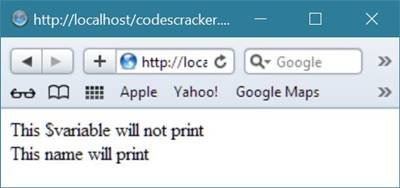Either you can place your string in single quote or in double quote. Let's take an example to understand about both singly-quoted string and doubly-quoted string in PHP.

```<html>
<title>PHP String Data Type - CodesCracker</title>
<body>
<?php
# singly-quoted string example - codescacker
\$singly_quoted_string = 'This string is in single quote';
var_dump(\$singly_quoted_string);
echo "<hr/>";
# doubly-quoted string example - codescacker
\$strvar1 = "Testing1";
\$strvar2 = [ "Testing2", "Testing3", "Testing4" ];
\$doubly_quoted_string = "This string is in double quote<br/>";
\$doubly_quoted_string .= "\$strvar1<br/>";
\$doubly_quoted_string .= "\$strvar2<br/>";
\$doubly_quoted_string .= "\$strvar2<br/>";
\$doubly_quoted_string .= "\$strvar2<br/>";
echo \$doubly_quoted_string;
?>
</body>
</html>```

Here is the sample output produced by the above PHP string data type example code: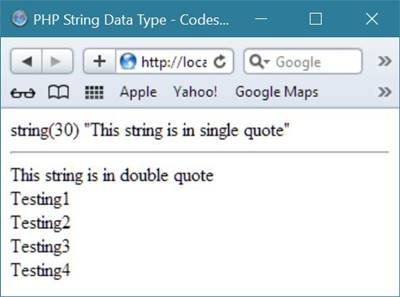## PHP Here Document

Using PHP here document, you are able to assign multiple lines to a single string vaeiable. Here is an example:

```<!DOCTYPE html>
<html>
<body>

<?php
\$channel =<<<_XML_
<channel>
<title>Here Document Example<title>
<description>PHP Tutorials from beginner to advance.</description>
</channel>
_XML_;

echo <<<END
This uses the "here document" syntax to output
multiple lines with variable interpolation. Note
that the here document terminator must appear on a
line with just a semicolon. no extra whitespace!
<br />
END;

print \$channel;
?>

</body>
</html>```

It will display the following result: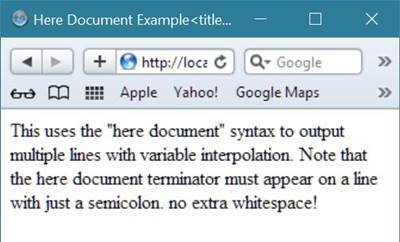## PHP Type Comparison

There are two type of type comparison available in PHP.

• loose comparison with ==
• strict comparison with ===

Here The strict comparison (===) in PHP ensures that the type and the value of both side of operator are the same.

## PHP Type Comparison Example

Here is an example of PHP type comparison, that is loose comparison (==) in PHP and strict comparison (===) in PHP example.

```<html>
<title>PHP Type Comparison - CodesCracker</title>
<body>
<p>PHP Loose (==) and Strict (===) Type Comparison Example</p>
<?php
// loose comparison (==) example in PHP
\$chck = var_dump(1 == 1);
echo \$chck;
echo "<br/>";
\$chck = var_dump(1 == "1");
echo \$chck;
echo "<br/>";
\$chck = var_dump(1 == true);
echo \$chck;
echo "<br/>";
\$chck = var_dump(0 == false);
echo \$chck;
echo "<hr/>";
# strict comparison (===) example in PHP
\$chck = var_dump(1 === 1);
echo \$chck;
echo "<br/>";
\$chck = var_dump(1 === "1");
echo \$chck;
echo "<br/>";
\$chck = var_dump(1 === true);
echo \$chck;
echo "<br/>";
\$chck = var_dump(0 === false);
echo \$chck;
echo "<hr/>";
?>
</body>
</html>```

Here is the sample output of the above PHP type comparison example code: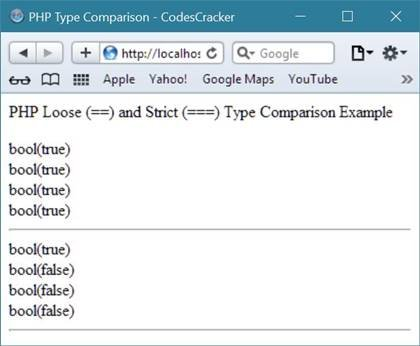## PHP Type Casting

Type casting in PHP is a manual process to force the data to convert into the required type.

Let's take an example of type casting in PHP to understand about type casting in PHP:

```<html>
<title>PHP Type Casting - CodesCracker</title>
<body>
<?php
# PHP Type Casting Example - CodesCracker
\$booln = true;
echo var_dump(\$booln);
echo "<br/>";
\$booln = false;
echo var_dump(\$booln);
echo "<br/>";
\$intgr = (int) true;
echo var_dump(\$intgr);
echo "<br/>";
\$intgr = (int) false;
echo var_dump(\$intgr);
echo "<br/>";
\$strng = (string) true;
echo var_dump(\$strng);
echo "<br/>";
\$strng = (string) false;
echo var_dump(\$strng);
echo "<br/>";
\$flt = (float) true;
echo var_dump(\$flt);
echo "<br/>";
\$flt = (float) false;
echo var_dump(\$flt);
echo "<br/>";
\$strng = "string";
echo var_dump((unset)\$strng);
echo "<br/>";
?>
</body>
</html>```

Here is the sample output of the above type casting example code in PHP: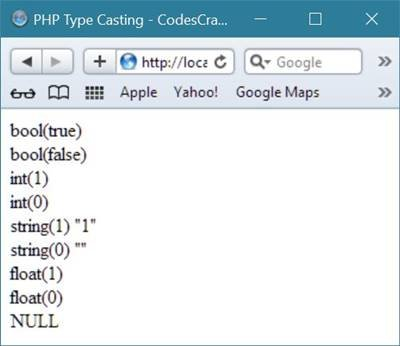Tools
Calculator